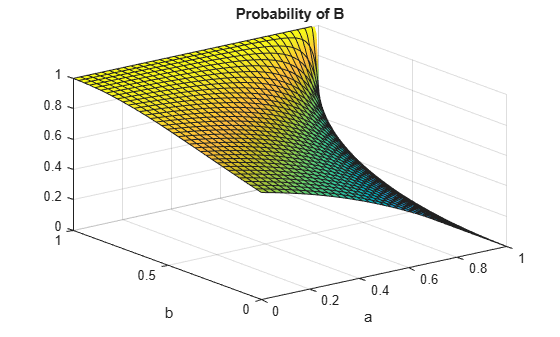# Markov Chain Analysis and Stationary Distribution

This example shows how to derive the symbolic stationary distribution of a trivial Markov chain by computing its eigen decomposition.

The stationary distribution represents the limiting, time-independent, distribution of the states for a Markov process as the number of steps or transitions increase.Define (positive) transition probabilities between states `A` through `F` as shown in the above image.

`syms a b c d e f cCA cCB positive;`

Add further assumptions bounding the transition probabilities. This will be helpful in selecting desirable stationary distributions later.

`assumeAlso([a, b, c, e, f, cCA, cCB] < 1 & d == 1);`

Define the transition matrix. States `A` through `F` are mapped to the columns and rows `1` through `6`. Note the values in each row sum up to one.

```P = sym(zeros(6,6)); P(1,1:2) = [a 1-a]; P(2,1:2) = [1-b b]; P(3,1:4) = [cCA cCB c (1-cCA-cCB-c)]; P(4,4) = d; P(5,5:6) = [e 1-e]; P(6,5:6) = [1-f f]; P```
```P =  $\left(\begin{array}{cccccc}a& 1-a& 0& 0& 0& 0\\ 1-b& b& 0& 0& 0& 0\\ \mathrm{cCA}& \mathrm{cCB}& c& 1-\mathrm{cCA}-\mathrm{cCB}-c& 0& 0\\ 0& 0& 0& d& 0& 0\\ 0& 0& 0& 0& e& 1-e\\ 0& 0& 0& 0& 1-f& f\end{array}\right)$```

Compute all possible analytical stationary distributions of the states of the Markov chain. This is the problem of extracting eigenvectors with corresponding eigenvalues that can be equal to 1 for some value of the transition probabilities.

`[V,D] = eig(P');`

Analytical eigenvectors

`V`
```V =  ```

Analytical eigenvalues

`diag(D)`
```ans =  $\left(\begin{array}{c}1\\ 1\\ c\\ d\\ a+b-1\\ e+f-1\end{array}\right)$```

Find eigenvalues that are exactly equal to 1. If there is any ambiguity in determining this condition for any eigenvalue, stop with an error - this way we are sure that below list of indices is reliable when this step is successful.

```ix = find(isAlways(diag(D) == 1,'Unknown','error')); diag(D(ix,ix))```
```ans =  $\left(\begin{array}{c}1\\ 1\\ d\end{array}\right)$```

Extract the analytical stationary distributions. The eigenvectors are normalized with the 1-norm or `sum(abs(X))` prior to display`.`

```for k = ix' V(:,k) = simplify(V(:,k)/norm(V(:,k)),1); end Probability = V(:,ix)```
```Probability =  ```

The probability of the steady state being `A` or `B` in the first eigenvector case is a function of the transition probabilities `a` and `b`. Visualize this dependency.

```fsurf(Probability(1), [0 1 0 1]); xlabel a ylabel b title('Probability of A');``````figure(2); fsurf(Probability(2), [0 1 0 1]); xlabel a ylabel b title('Probability of B');```The stationary distributions confirm the following (Recall states `A` through `F` correspond to row indices `1` through `6` ):

• State `C` is never reached and is therefore transient i.e. the third row is entirely zero.

• The rest of the states form three groups, { `A` , `B` }, { `D` } and { `E` , `F` } that do not communicate with each other and are recurrent.

#### Mathematical Modeling with Symbolic Math Toolbox

Get examples and videos